Bitcoin Forum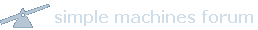April 16, 2021, 02:11:52 AM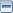Welcome, Guest. Please login or register.
 News: Latest Bitcoin Core release: 0.21.0 [Torrent]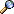Home Help Search Login Register More
 Pages:Author Topic: x^3+7=0 ?  (Read 1179 times)
Yves Cuicui
Newbie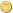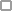Offline

Activity: 18
Merit: 0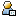September 03, 2013, 05:16:16 PM

Given the x coordinate of a point on the EC curve, it's easy to compute one of the y coordinate.

But, given y, how can we get x? In particular does someone know a solution to x^3+7 = 0 on the secp256k1 curve?

Thanks16185391121618539112

1618539112
 Report to moderator
16185391121618539112

1618539112
 Report to moderator
Advertised sites are not endorsed by the Bitcoin Forum. They may be unsafe, untrustworthy, or illegal in your jurisdiction. Advertise here.
16185391121618539112

1618539112
 Report to moderator
16185391121618539112

1618539112
 Report to moderator
mustyoshi
Sr. MemberOffline

Activity: 287
Merit: 250September 04, 2013, 01:24:49 AM

It only becomes trivial to crack the equation when the same "random" number is used in subsequent transactions. Basically the same way they got into Sony's servers, it can, and has been executed on the Blockchain, a few months back some application was using the same random numbers in transactions and people were able to calculate the private key.Yves Cuicui
NewbieOffline

Activity: 18
Merit: 0September 04, 2013, 10:49:05 AM

Thanks for your comment mustyoshi
My question is simply, what are the points that lies on the x axis.
Alternatively, how many are there, 1 or 3?johoe
Full MemberOffline

Activity: 217
Merit: 160September 04, 2013, 11:05:29 AM

It shouldn't be too difficult to compute the cubic-root modulo p. There are at most three solution, but I'm not sure if there are always three solutions.

The idea for square root is the following.  For every number x^p = x (mod p), hence x^(p+1) = x^2 (mod p), hence x^((p+1)/2) = x (mod p) and x^((p+1)/4) = sqrt(x) (mod p) is one of the two square roots.  The other is obtained by negation.

For cubic roots a similar approach should work.  If (p+1) is divisible by three then x^(p+1)/6 is a cubic root (I'm too lazy to check if this is the case).  There should be a general way to find a cubic root for any prime p.Donations to 1CF62UFWXiKqFUmgQMUby9DpEW5LXjypU3
jackjack
LegendaryOffline

Activity: 1176
Merit: 1079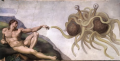May Bitcoin be touched by his Noodly AppendageSeptember 04, 2013, 11:06:44 AM

It shouldn't be too difficult to compute the cubic-root modulo p. There are at most three solution, but I'm not sure if there are always three solutions.

The idea for square root is the following.  For every number x^p = x (mod p), hence x^(p+1) = x^2 (mod p), hence x^((p+1)/2) = x (mod p) and x^((p+1)/4) = sqrt(x) (mod p) is one of the two square roots.  The other is obtained by negation.

For cubic roots a similar approach should work.  If (p+1) is divisible by three then x^(p+1)/6 is a cubic root (I'm too lazy to check if this is the case).  There should be a general way to find a cubic root for any prime p.

I tried yesterday but no, p is not divisible by three
It's divisible by 2, 4, 8, 16 then big numbers (>1M)Own address: 19QkqAza7BHFTuoz9N8UQkryP4E9jHo4N3 - Pywallet support: 1AQDfx22pKGgXnUZFL1e4UKos3QqvRzNh5 - Bitcointalk++ script support: 1Pxeccscj1ygseTdSV1qUqQCanp2B2NMM2
Pywallet: instructions. Encrypted wallet support, export/import keys/addresses, backup wallets, export/import CSV data from/into wallet, merge wallets, delete/import addresses and transactions, recover altcoins sent to bitcoin addresses, sign/verify messages and files with Bitcoin addresses, recover deleted wallets, etc.
fpgaminer
Hero MemberOffline

Activity: 560
Merit: 505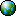September 04, 2013, 11:12:53 AM

Quote
But, given y, how can we get x? In particular does someone know a solution to x^3+7 = 0 on the secp256k1 curve?
Well, it's just a finite field, so this should work:

Code:
x = modular_cube_root ((y^2 - 7) % p, p)

I used the code listed here to solve your particular example.  It returns None, so probably there isn't an x that solves the equation when y is 0.Yves Cuicui
NewbieOffline

Activity: 18
Merit: 0September 04, 2013, 11:37:24 AMLast edit: September 04, 2013, 12:59:20 PM by Yves Cuicui

Thanks for these ideas. I will dig in.

Quote
I used the code listed here to solve your particular example.  It returns None, so probably there isn't an x that solves the equation when y is 0.

As N is odd and all points are duals (x,y) and (x,-y), there is at least one point (x,0), so you must get one.johoe
Full MemberOffline

Activity: 217
Merit: 160September 04, 2013, 01:30:39 PM

There is always the point at infinity (the neutral element of the elliptic curve group).  This explains why the total number of points is odd if there is no solution for y=0.Donations to 1CF62UFWXiKqFUmgQMUby9DpEW5LXjypU3
Yves Cuicui
NewbieOffline

Activity: 18
Merit: 0September 04, 2013, 02:20:51 PM

Shame on me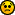Yves Cuicui
NewbieOffline

Activity: 18
Merit: 0February 23, 2014, 10:07:16 PM

This just to finalize this topic.

Because P=9xu+7, if a cubic root exists it can be computed by r1=a^((P+2)/9).
The other two solutions are:
r2=0x3fffffffffffffffffffffffffffffffffffffffffffffffffffffffbfffff0c . r1
r3=0x1c71c71c71c71c71c71c71c71c71c71c71c71c71c71c71c71c71c71c555554e9 . r1

Then it is easy to see that -7 has no cubic root because ((-7)^((P+2)/9))^3 <> -7

Then there is no points with y=0

my 2 cents
Thanks to you allPages: 
Jump to: